[TOP]

Chemical kinetics for various processes (I):

(1) Enzyme Reactions - The Michaelis-Menten mechanism - an example of consecutive elementary reactions

[ TOP ]

(1) Enzyme Reactions - The Michaelis-Menten mechanism - an example of consecutive elementary reactions

The Michaelis-Menten mechanism is an example of a reaction involving an intermediate. We shall consider a general case where a substrate S is converted into products P (catalysed by the enzyme E) through the following mechanism:where ES denotes the substrate/enzyme intermediate, see fig. 1.Fig. 1: The basis of the Michaelis-Menten mechanism of enzyme action. Only a fragment of the large enzyme molecule E is shown.

This means that the rate of product formation is given by:where is we assume a pre-equilibrium and apply the steady state approximation we get: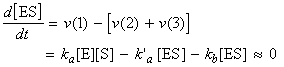i.e.:Also, because the enzyme is not being consumed in the reaction, we may conclude that the total concentration of the enzyme is given by (assuming that the reaction volume remains constant):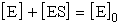i.e.: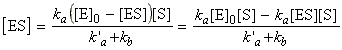i.e.:i.e.:i.e.: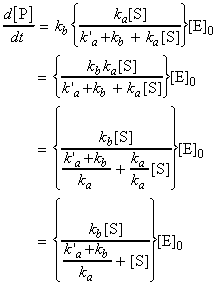i.e. the rate of formation of the product may be expressed in terms of the effective rate constant, k, as: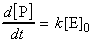where (see figure 2a):where KM is the Michaelis constant, given by: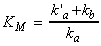According to these equations:
1. The rate of enzymolysis varies linearly with the initial enzyme concentration;
2. The rate of enzymolysis varies in a more complicated manner with the substrate concentration (see plot of k/kbvs. [S] / KM )
The values of KM and kb may be obtained by inverting the equation: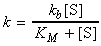to give the Lineweaver-Burk equation: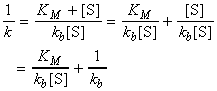A plot of 1/k vs. 1/[S] (Lineweaver-Burk plot) which gives 1/kb as intercept, and KM/kb as gradient. (see fig. 2b.)(a)(b)

Fig. 2: (a) The variation of the effective rate constant k with substrate concentration according to the Michaelis-Menten mechanism.(b) A Lineweaver-Burk plot for the analysis of an enzymolysis that proceeds by a Michaelis-Menten mechanism, and the significance of the intercepts and the slope.

>>> Let is now consider the scenarios

(A) When [S] >> KM :

The rate of enzymolysis is given by: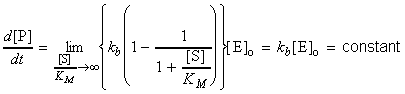This means that:
• The rate becomes zero-order in S.
• Under these conditions, the rate is constant (i.e. There is so much S present, that it remains at effectively the same concentration, even though products are forming.
• The rate of formation is at a maximum, and (1) kb / [E]0 is known as the maximum velocity, and kb is known as the maximum turnover number.

• (B) When, [S] is small, i.e. [S] << KM

In such cases, we make use of: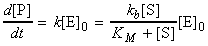and assume that KM + [S] is approximately KM, i.e.:i.e. the rate is proportional to both [S] and [E]0.